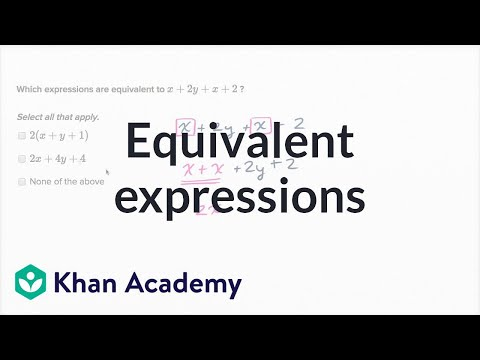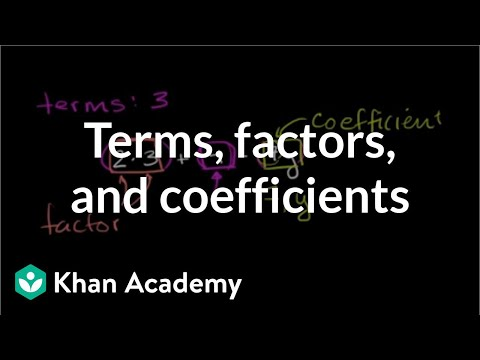Video

# Expression value intuition (Full video)

Description: Explore how the values of algebraic expressions like 100-x, 5/x + 5, and (3y)/(2y) change as the values of the variables change. We wanna know how does this expression change as x increases? You say, "Okay, I have 100 and I'm subtracting x." So, as x increases, as x increases over here, I'm going to be subtracting larger and larger values.

### Other videos you might be interested in### Equivalent expressions (Full video)